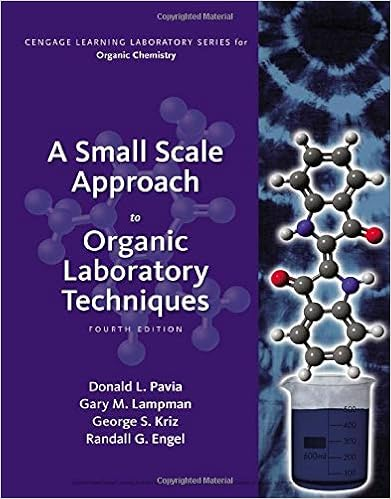1 what is the charge on the iron ion in the starting

• Lab Report
• 6

This preview shows page 2 - 4 out of 6 pages.

We have textbook solutions for you!
The document you are viewing contains questions related to this textbook.The document you are viewing contains questions related to this textbook.
Chapter T19 / Exercise 5
A Small Scale Approach to Organic Laboratory Techniques
Lampman/PaviaExpert Verified
1. What is the charge on the Iron Ion in the starting material, Ferrous Ammonium Sulfate Hexahydrate. Similarly, what is the charge on the Iron Ion in the product compound, Potassium Ferric Oxalate Tri- hydrate? How about for the reaction intermediate FeC 2 O 4* 2H 2 O.
2. In this laboratory you have used two different types of filtration. One is Gravity Filtration. The other is filtration using a Buchner Funnel. What is the principle difference in the purpose of these types of filtra- tion?
3. Suppose I expect a Loss of 40%, how much Ferrous Ammonium Sulfate Hexahydrate must I start with in order to obtain 100g of Potassium Ferric Oxalate Trihydrate?
4. Ammonium Sulfate ((NH4)2SO4), Sulfuric Acid (H2SO4) and Water (H2O) are all by- products of the first reaction in our synthesis. How much (mass) of each are produced when starting 5.00g of Ferrous Ammonium Sulfate Hexahydrate?
5. What ions are formed when each of the following Water Soluble coordination compounds dissolves in Water:
We have textbook solutions for you!
The document you are viewing contains questions related to this textbook.The document you are viewing contains questions related to this textbook.
Chapter T19 / Exercise 5
A Small Scale Approach to Organic Laboratory Techniques
Lampman/PaviaExpert Verified
The Determination of Oxalate Ion in Ferric Oxalate Trihydrate Using Titrimetry The mass percentage of Oxalate Ion present in our sample was found by using stoichiometry, the volume of Permanganate Ion solution required for complete reaction, and the concentrate of the solution used. The concentration of the Permanganate Ion Solution was found to be .008665M. The percentage of Ox- alate Ion present in our sample was found to be about 55%.
•••